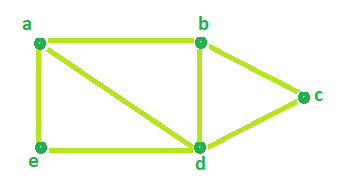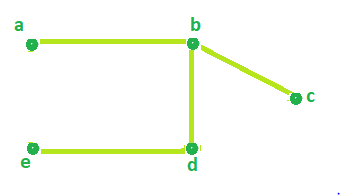# Program to find Circuit Rank of an Undirected Graph

Given the number of Vertices and the number of Edges of an Undirected Graph. The task is to determine the Circuit rank.

Circuit Rank: The Circuit rank of an undirected graph is defined as the minimum number of edges that must be removed from the graph to break all of its cycles, converting it into a tree or forest.

Examples:

```Input : Edges = 7 , Vertices = 5
Output : Circuit rank = 3

Input : Edges = 7 , Vertices = 6
Output : Circuit rank = 2
```

## Recommended: Please try your approach on {IDE} first, before moving on to the solution.

Formula:

```Circuit rank = Edges - (Vertices - 1)
```

## Recommended: Please try your approach on {IDE} first, before moving on to the solution.

Look at the sample graph below,Total number of Edges = 7 and Vertices = 5.

According to the above formula,

```Circuit Rank =  Edges - (Vertices - 1)
=  7 - (5 - 1)
= 3
```

Therefore, Circuit Rank of the above graph = 3.

It can be seen in the below image that by removing 3 edges(a-d, a-e, c-d) from the above graph, all of it cycles can be removed.Below is the implementation of the above approach:

## C++

 `// C++ Program to find Circuit Rank of an Undirected Graph ` `#include ` `using` `namespace` `std; ` ` `  `// Function that calculates the ` `// Circuit rank of the Graph. ` `int` `Rank(``int` `Edges, ``int` `Vertices) ` `{ ` `    ``int` `result = 0; ` ` `  `    ``// calculates Circuit Rank ` `    ``result = Edges - Vertices + 1; ` ` `  `    ``return` `result; ` `} ` ` `  `// Driver Code ` `int` `main() ` `{ ` `    ``int` `Edges = 7, Vertices = 5; ` ` `  `    ``cout << ``"Circuit Rank = "` `<< Rank(Edges, Vertices); ` ` `  `    ``return` `0; ` `} `

## Java

 `//Java Program to find Circuit Rank of an Undirected Graph ` ` `  `public` `class` `GFG { ` ` `  `    ``//Function that calculates the ` `    ``//Circuit rank of the Graph. ` `    ``static` `int` `Rank(``int` `Edges, ``int` `Vertices) ` `    ``{ ` `     ``int` `result = ``0``; ` ` `  `     ``// calculates Circuit Rank ` `     ``result = Edges - Vertices + ``1``; ` ` `  `     ``return` `result; ` `    ``} ` ` `  `    ``//Driver Code ` `    ``public` `static` `void` `main(String[] args) { ` `         `  `         ``int` `Edges = ``7``, Vertices = ``5``; ` ` `  `         ``System.out.println(``"Circuit Rank = "` `+ Rank(Edges, Vertices)); ` `    ``} ` `} `

## Python 3

 `# Python 3 program to find Circuit Rank of  ` `# an Undirected Graph ` ` `  `# Function that calculates the  ` `# Circuit rank of the Graph.  ` `def` `Rank(Edges, Vertices) : ` ` `  `    ``# calculates Circuit Rank ` `    ``result ``=` `Edges ``-` `Vertices ``+` `1` ` `  `    ``return` `result ` ` `  `# Driver code      ` `if` `__name__ ``=``=` `"__main__"` `: ` ` `  `    ``Edges, Vertices ``=` `7``, ``5` ` `  `    ``print``(``"Circuit Rank ="``,Rank(Edges, Vertices)) ` ` `  ` `  `# This code is contributed by ANKITRAI1 `

## C#

 `// C# Program to find Circuit ` `// Rank of an Undirected Graph ` `using` `System; ` ` `  `class` `GFG  ` `{ ` ` `  `// Function that calculates the ` `// Circuit rank of the Graph. ` `static` `int` `Rank(``int` `Edges, ` `                ``int` `Vertices) ` `{ ` `    ``int` `result = 0; ` `     `  `    ``// calculates Circuit Rank ` `    ``result = Edges - Vertices + 1; ` `     `  `    ``return` `result; ` `} ` ` `  `// Driver Code ` `public` `static` `void` `Main() ` `{ ` `    ``int` `Edges = 7, Vertices = 5; ` ` `  `    ``Console.WriteLine(``"Circuit Rank = "` `+  ` `                       ``Rank(Edges, Vertices)); ` `} ` `} ` ` `  `// This code is contributed  ` `// by inder_verma `

## PHP

 ` `

Output:

```Circuit Rank = 3
```

My Personal Notes arrow_drop_upCheck out this Author's contributed articles.

If you like GeeksforGeeks and would like to contribute, you can also write an article using contribute.geeksforgeeks.org or mail your article to contribute@geeksforgeeks.org. See your article appearing on the GeeksforGeeks main page and help other Geeks.

Please Improve this article if you find anything incorrect by clicking on the "Improve Article" button below.

Article Tags :
Practice Tags :

Be the First to upvote.

Please write to us at contribute@geeksforgeeks.org to report any issue with the above content.